# DAV Class 8 Maths Chapter 1 Brain Teasers Solutions

The DAV Class 8 Maths Book Solutions and DAV Class 8 Maths Chapter 1 Brain Teasers Solutions of Squares and Square Roots offer comprehensive answers to textbook questions.

## DAV Class 8 Maths Ch 1 Brain Teasers Solutions

Question 1A.
Tick (✓) the correct option.

(i) The difference between the squares of two consecutive numbers is equal to their
(a) difference
(b) sum
(c) product
(d) quotient
Solution:
(b) sum

(ii) What will be the digit in the thousands place of (1111)2 ?
(a) 3
(b) 4
(c) 2
(d) 1
Solution:
(b) 4
[(1111)2 = 1111 × 1111 = 1234321]

(iii) Perfect squares cannot have 2, 3, ___ and ___ in its ones place.
(a) 1, 7
(b) 5, 6
(c) 7, 8
(d) 7, 9
Solution:
(c) 7, 8

(iv) The smallest number by which 72 must be divided to make it a perfect square is
(a) 4
(b) 5
(c) 3
(d) 2
Solution:
(d) 2
[72 = 2 × 2 × 2 × 3 × 3]

(v) The square root of 3.052009 has ___ decimal places.
(a) 3
(b)4
(c) 5
(d) 1
Solution:
(a) 3
[$$\sqrt{3.052009}$$= 1.747]Question 1B.

(i) How many non-square numbers are there between 132 and 142 ?
(ii) Write the first four triangular numbers.
(iii) Is 5, 7, 9 a Pythagorean triplets? Why? Justify.
(iv) Find √9 by repeated subtraction method.
(v) Find the measure of the side of a square handkerchief of area 324 cm2.
Solution:
(i) There are twenty-six non-square numbers between 132 and 142 (i.e. 2 × 13 = 26).
(ii) First four triangular numbers are 1, 3, 6 and 10.
(iii) 5, 7, 9 is not a Pythagorian triplet as 52 + 72 ≠ 92.
(iv) Using repeated subtraction method, we have
Step 1: 9 – 1 = 8
Step 2: 8 – 3 = 5
Step 3: 5 – 5 = 0
We have subtracted 1, 3 and 5, the successive odd numbers from 9 and obtained 0.

Question 2.
Find the square root of 10, correct to four places of decimal.
Solution: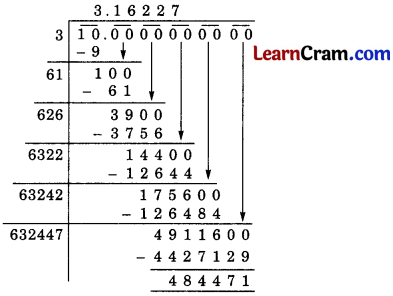$$\sqrt{10}$$ = 3.16227 = 3.163.Question 3.
Find the values of $$\sqrt{3.1428}$$ and $$\sqrt{0.31428}$$ correct to three decimal places.
Solution:$$\sqrt{3.1428}$$ = 1.7727 = 1.773$$\sqrt{0.31428}$$ = 0.5606 = 0.561

Question 4.
Simplify :
(i) $$\frac{\sqrt{0.0441}}{\sqrt{0.000441}}$$
(ii) $$\sqrt{49}+\sqrt{0.49}+\sqrt{0.0049}$$
Solution:
(i) $$\frac{\sqrt{0.0441}}{\sqrt{0.000441}}$$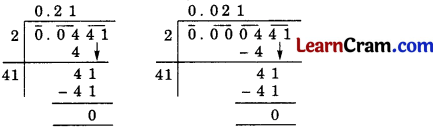$$\frac{\sqrt{0.0441}}{\sqrt{0.000441}}=\frac{0.21}{0.021}$$ = 10

(ii) $$\sqrt{49}+\sqrt{0.49}+\sqrt{0.0049}$$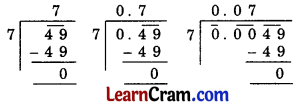= 7 + 0.7 + 0.07 = 7.77Question 5.
The area of a square field is 101 $$\frac{1}{400}$$ m2. Find the length of one side of the field.
Solution:
101 $$\frac{1}{400}$$ = $$\frac{40401}{400}$$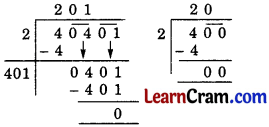Side of the square field = $$\sqrt{\frac{40401}{400}}$$
= $$\frac{201}{20}=10 \frac{1}{20}$$ m.

Question 6.
What is that number which when multiplied by itself gives 227.798649?
Solution:
Let the required decimal be x
x × x = 227.798649
x2 = 227.798649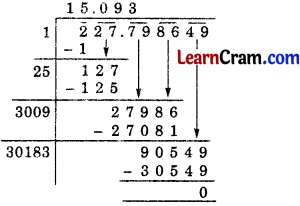∴ x = $$\sqrt{227.798649}$$ = 15.093
Hence the required decimal = 15.093.Question 7.
In a lecture hall, 8649 students are sitting in such a manner that there are as many students in a row as there are rows in the lecture hail. How many students are there in each row of the lecture hall?
Solution:
Let the number of students in lecture hail be x
∴ Number of rows = x
Total number of students = x × x = x2
:. x2 = 8649x = $$\sqrt{8649}$$ = 93.
Hence the required number of students = 93.

Question 8.
A General wishing to draw up his 64019 men in the form of a square found that he had 10 men extra. Find the numebr of men in the front row.
Solution:
Number of men = 64019
Number of extra men = 10
∴ Number of remaining men = 64019 – 10 = 64009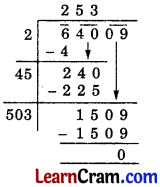Number of men in front row = $$\sqrt{64009}$$ = 253
Hence the required number of men = 253.### DAV Class 8 Maths Chapter 1 HOTS

Question 1.
The cost of levelling a square lawn at 15 per square metre is 19,935. Find the cost of fencing the lawn at 22 per metre.
Solution:
Total cost of levelling = 19,935
Cost of levelling per square metre = 15
∴ Area of square lawn = $$\frac{19,935}{15}$$ = 1329
Side of square lawn = $$\sqrt{1329}$$ = 36.455
Perimeter of square lawn = 36.455 × 4 = 145.82
Thus, the cost of fencing the lawn at 22 per metre = 145.82 × 22 = 3208.04.

Question 2.
If √2 = 1.414, √5 = 2.236 and √3 = 1.732, find the value of:
(i) $$\sqrt{72}+\sqrt{48}$$

(ii) $$\sqrt{\frac{125}{64}}$$
Solution:
(i) $$\sqrt{72}+\sqrt{48}=\sqrt{2 \times 2 \times 2 \times 3 \times 3}+\sqrt{2 \times 2 \times 2 \times 2 \times 3}$$
= 2 × 3 × √2 + 2 × 2 × √3
= 6√2 + 4√3
= 6 × 1.414 + 4 × 1.732 (Given √2 = 1.414 and √3 = 1.732)
= 8.484 + 6.928
= 15.412.

(ii) $$\sqrt{\frac{125}{64}}=\sqrt{\frac{5 \times 5 \times 5}{2 \times 2 \times 2 \times 2 \times 2 \times 2}}$$
= $$\frac{5 \times \sqrt{5}}{2 \times 2 \times 2}=\frac{5 \times 2.236}{8}$$
= $$\frac{11.18}{8}$$ = 1.3975.### DAV Class 8 Maths Chapter 1 Enrichment Questions

Question 1.
The product of two numbers is 1296. If one number is 16 times the other, find the number.
Solution:
Let the other number be x.
Then, the first number = 16x
Now, according to question,
16x × x = 1296
⇒ x2 = $$\frac{1296}{16}$$
⇒ x2 = 81
∴ x = $$\sqrt{81}$$ = 9
⇒ other number = 9
and first number = 16 × 9 = 144
Thus, the required numbers are 144 and 9.Question 2.
Find the value of $$\sqrt{50625}$$ and hence the value of $$\sqrt{506.25}$$ + $$\sqrt{5.0625}$$.
Solution:
$$\sqrt{50625}$$ = $$\sqrt{3 \times 3 \times 3 \times 3 \times 5 \times 5 \times 5 \times 5}$$
= 3 × 3 × 5 × 5 = 225
Now,
$$\sqrt{506.25}$$ + $$\sqrt{5.0625}$$ = $$\sqrt{\frac{50625}{100}}+\sqrt{\frac{50625}{10000}}$$= $$\sqrt{\left(\frac{225}{10}\right)^2}+\sqrt{\left(\frac{225}{100}\right)^2}$$
= $$\frac{225}{10}+\frac{225}{100}$$
= 22.5 + 2.5 = 24.75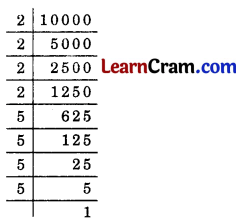∴ $$\sqrt{10000}$$ = 2 × 2 × 5 × 5 = 100
∴ $$\sqrt{100}$$ = 2 × 5 = 10

Question 3.
Write a Pythagorean triplet if one number is 14.
Solution:
Let the smallest number be 14, so that
2m = 14
⇒ m = $$\frac{14}{2}$$ = 7.
Now, m2 – 1 = 72 – 1
= 49 – 1 = 48 and,
m2 + 1 = 72 + 1
= 49 + 1 = 50.
Thus, 14, 48 and 50 are the Pythagorian triplet.Question 1.
Find $$\sqrt{361 \times 225}$$.
Solution:
$$\sqrt{361 \times 225}=\sqrt{361} \times \sqrt{225}$$
[∵ $$\sqrt{}$$ = √a × √b]
= 19 × 15 = 285

Question 2.
Find $$\sqrt{1 \frac{56}{169}}$$.
Solution:
$$\sqrt{1 \frac{56}{169}}=\sqrt{\frac{225}{169}}=\sqrt{\frac{225}{169}}=\frac{15}{13}$$

Question 3.
Find $$\sqrt{4096}$$.
Solution:
$$\sqrt{4096}$$ = 64Question 4.
Find $$\sqrt{17.64}$$
Solution:
$$\sqrt{17.64}$$ = 4.2Question 5.
Find $$\sqrt{0.119025}$$
Solution:
$$\sqrt{0.119025}$$ = 0.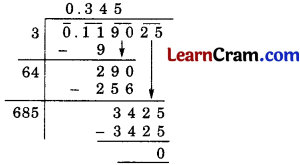Question 6.
Find the square root of 7 upto three places of decimals.
Solution:
√7 = 2.645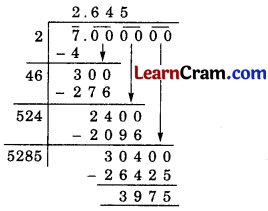Question 7.
Find square root of 0.1 upto three places of decimals.
Solution:
$$\sqrt{0.1}$$ = 0.316.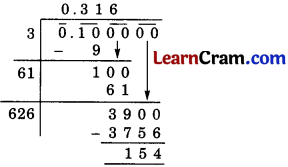Question 8.
Find the number of digits in the square root of the following numbers (without any calculation).
(i) 144
(ii) 390625
Solution:
(i) 144 has 3 digits (odd).
∴ Number of digits in its square root = $$\frac{n+1}{2}=\frac{3+1}{2}=\frac{4}{2}$$ = 2

(ii) 390625 has 6 digits (even)
∴ Number of digits in its square root = $$\frac{n}{2}=\frac{6}{2}$$ = 3.

Question 9.
Find the square root of 250 by estimation.
Solution:
The two nearest perfect square number to 250 are 225 and 256.
∴ 225 < 250 < 256 ⇒ 152 < 250 < 16
Let us try for 15.5.
15.5 × 15.5 = 240.25
∴ 15.52 < 250 < 16
Now 15.7 × 15.7 = 246.49
∴ 15.72 < 250 < 162
and 15.8 × 15.8 = 249.64
and 15.9 × 15.9 = 252.81
∴ 15.82 < 250 < 15.92
Hence, $$\sqrt{250}$$ = 15.85 (approximate)Question 10.
The area of a square plot is 1000 m2. Find the estimated length of the side of the plot.
Solution:
The nearest perfect square number to 1000 are 961 and 1024.
∴ 961 < 1000 < 1024
⇒ 312 < 1000 < 322
Let us try for 31.4.
31.4 × 31.4 = 985.96 and 31.8 × 31.8 = 1011.24
∴ 31.42 < 1000 < 31.82
31.62 < 1000 < 31.72
∴ Estimated value of $$\sqrt{1000}$$ = 31.65 m.

Question 11.
How many natural numbers lie between 252 and 262?
Solution:
Here n = 25 and n + 1 = 26
∴ Natural numbers between 252 and 262
= 25 + 26 = 51

Question 12.
Find square root of 11025 by prime factorisation.
Solution: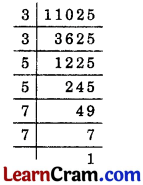11025 = 3 × 3 × 5 × 5 × 7 × 7
$$\sqrt{11025}$$ = 3 × 5 × 7 = 105

Question 13.
Find the value of $$\sqrt{\frac{243}{867}}$$ using prime factorisation method.
Solution: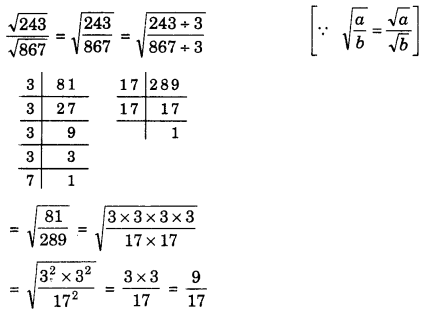Question 14.
Find the least number of six-digits which is a perfect square. Also, find the square root of this number.
Solution:
The least six-digit number is 100000.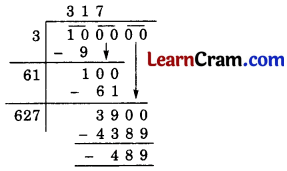Hence, the least six-digit perfect square number is
100000 + 489 = 100489 and $$\sqrt{100489}$$ = 317.

Question 15.
Find the square root of 147.1369.
Solution: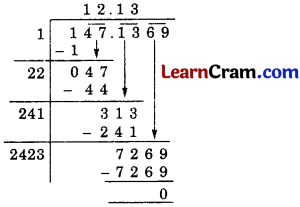Hence, $$\sqrt{147.1369}$$ = 12.13

Question 16.
Find the square root of 2$$\frac{1}{5}$$ correct to 3 places of decimal.
Solution:$$\sqrt{2 \frac{1}{5}}=\sqrt{\frac{11}{5}}=\sqrt{2.2}$$
∴ $$\sqrt{2.2}$$ = 1.483.Multiple Choice Questions:

Question 1.
How many natural numbers lie between 352 and 362?
(a) 70
(b) 71
(c) 37
(d) 34
Solution:
(b) 71

Question 2.
How many digits are there in the square root of 1234321?
(a) 3
(b) 5
(c) 4
(d) 6
Solution:
(c) 4

Question 3.
The number of non-perfect squares between 25 and 36 is
(a) 8
(b) 9
(c) 10
(d) 11
Solution:
(c) 10

Question 4.
The value of 1 + 3 + 5 + 7 + 9 + …………… is
(a) 122
(b) 112
(c) 102
(d) 132
Solution:
(b) 112Question 5.
The smallest number by which 9408 must be divided to get a perfect square is
(a) 4
(b) 5
(c) 3
(d) 1
Solution:
(c) 3

Question 6.
The square root of 1521 is
(a) 31
(b) 41
(c) 39
(d) 49
Solution:
(c) 39

Question 7.
The least number that must be added to 5607 to make the sum a perfect square is
(a) 18
(b) 17
(c) 19
(d) none of these
Solution:
(a) 18

Question 8.
The square root of 0.1 is
(a) 0.123
(b) 0.361
(c) 0.136
(d) none of these
Solution:
(d) none of these

Question 9.
The value of $$\sqrt{49}+\sqrt{0.49}$$ is
(a) 7.07
(b) 7.007
(c) 0.7
(d) 7.7
Solution:
(d) 7.7Question 10.
A perfect square number leaves 0 or 1 as remainder on division by
(a) 7
(b) 2
(c) 3
(d) 1
Solution:
(c) 3